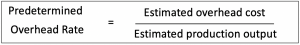Predetermined overhead rate is the estimated overhead that will allocate to each product at the begining of accounting period. It is equal to the estimate overhead divided by the estimate production quantity.

The company needs to use predetermined overhead rate to calculate the cost of goods sold and inventory balance. Cost of goods sold equal to the sales quantity multiply by the total cost per unit which include the overhead cost. The overhead cost per unit is the predetermined overhead rate. We also use the same rate to calculate the inventory balance at the end of accounitng period. However, the variance between actual overhead and estimated will be reconciled and adjust to the financial statement.

Many accountants always ask about specific time which we need to do this, at what point in time is the predetermined overhead rate calculated. The predetermined rate usually be calculated at the beginning of the accounting period by relying on the management experience and prior year data.## Example

Company A calculates the predetermined rate for its coming year. The company uses labor hours as the basis for allocation. The production manager has told us that the manufacturing overhead will be \$ 500,000 for the whole year and the company expected to spend 20,000 hours on direct labor. The management concern about how to find a predetermined overhead rate for costing.

Calculating predetermined overhead rate can be done as follow:

Predetermined overhead rate = \$ 500,000 / 20,000 hours = \$ 25 per direct labor

The product requires 2 hours of labor work so that it will require \$50 of overhead (\$25 * 2 hours). Based on this information the predetermined overhead rate is \$ 25 per labor hour.